# Predicting US house prices using Multiple Linear Regression in R

18 de Jul de 2019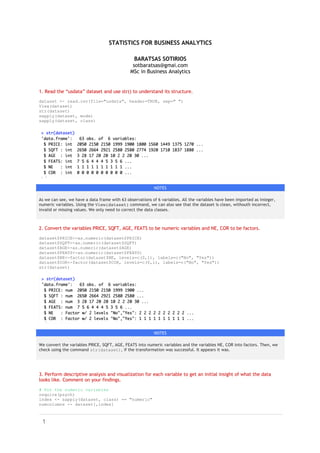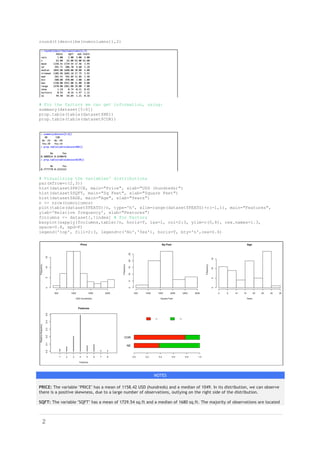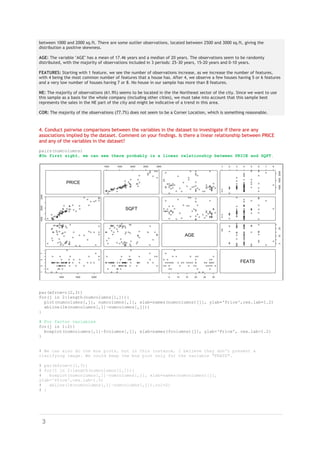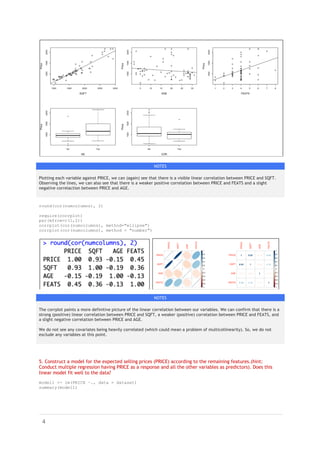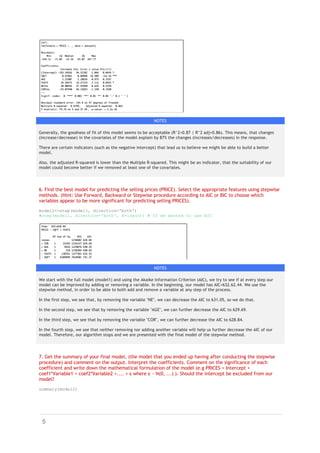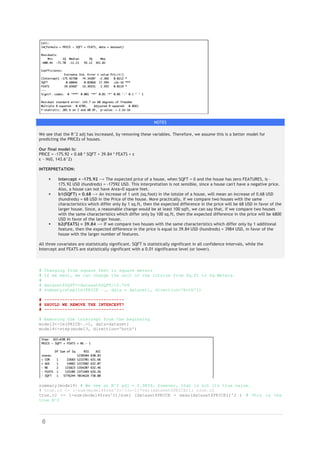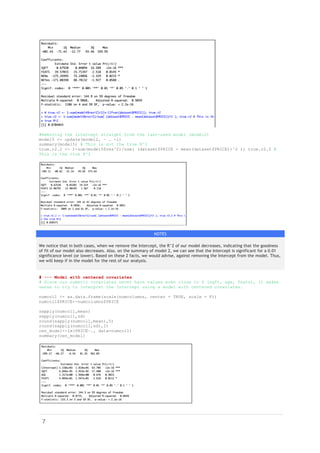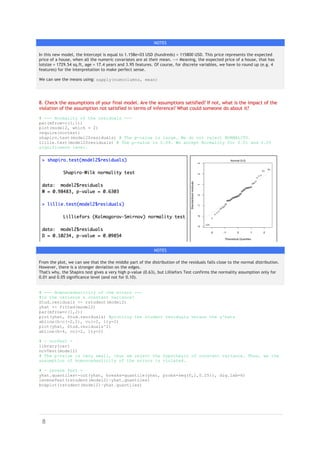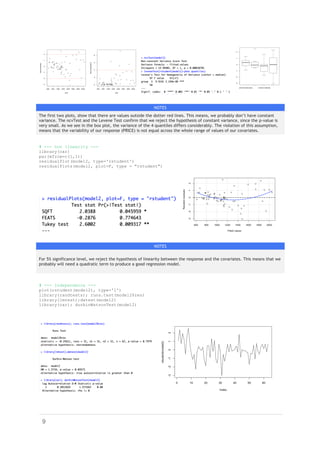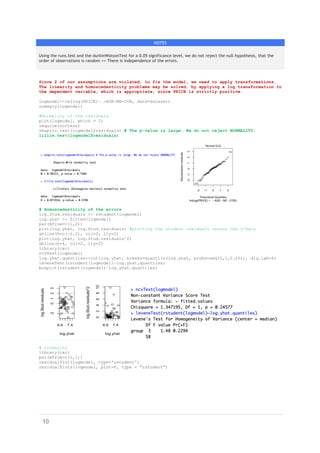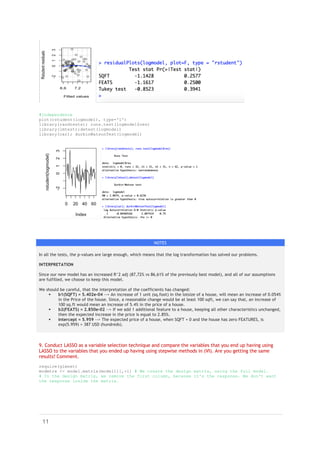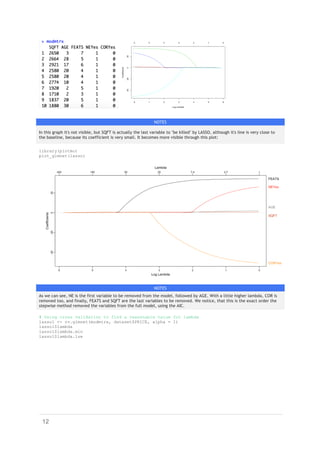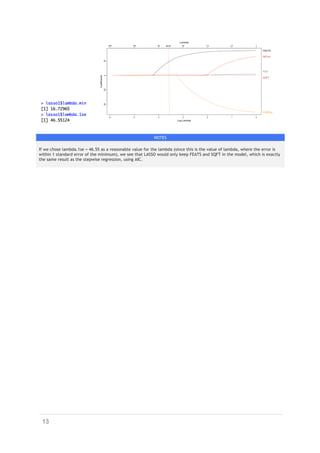Próximo SlideShareCorrelation analysis notes
Cargando en ... 3
1 de 13

### Predicting US house prices using Multiple Linear Regression in R

1. 1 STATISTICS FOR BUSINESS ANALYTICS BARATSAS SOTIRIOS sotbaratsas@gmai.com MSc in Business Analytics 1. Read the “usdata” dataset and use str() to understand its structure. dataset <- read.csv(file="usdata", header=TRUE, sep=" ") View(dataset) str(dataset) sapply(dataset, mode) sapply(dataset, class) NOTES As we can see, we have a data frame with 63 observations of 6 variables. All the variables have been imported as integer, numeric variables. Using the View(dataset) command, we can also see that the dataset is clean, withouth incorrect, invalid or missing values. We only need to correct the data classes. 2. Convert the variables PRICE, SQFT, AGE, FEATS to be numeric variables and NE, COR to be factors. dataset\$PRICE<-as.numeric(dataset\$PRICE) dataset\$SQFT<-as.numeric(dataset\$SQFT) dataset\$AGE<-as.numeric(dataset\$AGE) dataset\$FEATS<-as.numeric(dataset\$FEATS) dataset\$NE<-factor(dataset\$NE, levels=c(0,1), labels=c("No", "Yes")) dataset\$COR<-factor(dataset\$COR, levels=c(0,1), labels=c("No", "Yes")) str(dataset) NOTES We convert the variables PRICE, SQFT, AGE, FEATS into numeric variables and the variables NE, COR into factors. Then, we check using the command str(dataset), if the transformation was successful. It appears it was. 3. Perform descriptive analysis and visualization for each variable to get an initial insight of what the data looks like. Comment on your findings. # For the numeric variables require(psych) index <- sapply(dataset, class) == "numeric" numcolumns <- dataset[,index]
2. 2 round(t(describe(numcolumns)),2) # For the factors we can get information, using: summary(dataset[5:6]) prop.table(table(dataset\$NE)) prop.table(table(dataset\$COR)) # Visualizing the variables' distributions par(mfrow=c(2,3)) hist(dataset\$PRICE, main="Price", xlab="USD (hundreds)") hist(dataset\$SQFT, main="Sq Feet", xlab="Square Feet") hist(dataset\$AGE, main="Age", xlab="Years") n <- nrow(numcolumns) plot(table(dataset\$FEATS)/n, type='h', xlim=range(dataset\$FEATS)+c(-1,1), main="Features", ylab='Relative frequency', xlab="Features") fcolumns <- dataset[,!index] # for Factors barplot(sapply(fcolumns,table)/n, horiz=T, las=1, col=2:3, ylim=c(0,8), cex.names=1.3, space=0.8, xpd=F) legend('top', fill=2:3, legend=c('No','Yes'), horiz=T, bty='n',cex=0.6) NOTES PRICE: The variable "PRICE" has a mean of 1158.42 USD (hundreds) and a median of 1049. In its distribution, we can observe there is a positive skewness, due to a large number of observations, outlying on the right side of the distribution. SQFT: The variable "SQFT" has a mean of 1729.54 sq.ft and a median of 1680 sq.ft. The majority of observations are located
3. 3 between 1000 and 2000 sq.ft. There are some outlier observations, located between 2500 and 3000 sq.ft, giving the distribution a positive skewness. AGE: The variable "AGE" has a mean of 17.46 years and a median of 20 years. The observations seem to be randomly distributed, with the majority of observations included in 3 periods: 25-30 years, 15-20 years and 0-10 years. FEATURES: Starting with 1 feature, we see the number of observations increase, as we increase the number of features, with 4 being the most common number of features that a house has. After 4, we observe a few houses having 5 or 6 features and a very low number of houses having 7 or 8. No house in our sample has more than 8 features. NE: The majority of observations (61.9%) seems to be located in the the Northeast sector of the city. Since we want to use this sample as a basis for the whole company (including other cities), we must take into account that this sample best represents the sales in the NE part of the city and might be indicative of a trend in this area. COR: The majority of the observations (77.7%) does not seem to be a Corner Location, which is something reasonable. 4. Conduct pairwise comparisons between the variables in the dataset to investigate if there are any associations implied by the dataset. Comment on your findings. Is there a linear relationship between PRICE and any of the variables in the dataset? pairs(numcolumns) #On first sight, we can see there probably is a linear relationship between PRICE and SQFT. par(mfrow=c(2,3)) for(j in 2:length(numcolumns[1,])){ plot(numcolumns[,j], numcolumns[,1], xlab=names(numcolumns)[j], ylab='Price',cex.lab=1.2) abline(lm(numcolumns[,1]~numcolumns[,j])) } # For factor variables for(j in 1:2){ boxplot(numcolumns[,1]~fcolumns[,j], xlab=names(fcolumns)[j], ylab='Price', cex.lab=1.2) } # We can also do the box plots, but in this instance, I believe they don't present a clarifying image. We could keep the box plot only for the variable "FEATS". # par(mfrow=c(1,3)) # for(j in 2:length(numcolumns[1,])){ # boxplot(numcolumns[,1]~numcolumns[,j], xlab=names(numcolumns)[j], ylab='Price',cex.lab=1.5) # abline(lm(numcolumns[,1]~numcolumns[,j]),col=2) # }
4. 4 NOTES Plotting each variable against PRICE, we can (again) see that there is a visible linear correlation between PRICE and SQFT. Observing the lines, we can also see that there is a weaker positive correlation between PRICE and FEATS and a slight negative correlaction between PRICE and AGE. round(cor(numcolumns), 2) require(corrplot) par(mfrow=c(1,2)) corrplot(cor(numcolumns), method="ellipse") corrplot(cor(numcolumns), method = "number") NOTES The corrplot paints a more definitive picture of the linear correlation between our variables. We can confirm that there is a strong (positive) linear correlation between PRICE and SQFT, a weaker (positive) correlation between PRICE and FEATS, and a slight negative correlation between PRICE and AGE. We do not see any covariates being heavily correlated (which could mean a problem of multicollinearity). So, we do not exclude any variables at this point. 5. Construct a model for the expected selling prices (PRICE) according to the remaining features.(hint: Conduct multiple regression having PRICE as a response and all the other variables as predictors). Does this linear model fit well to the data? model1 <- lm(PRICE ~., data = dataset) summary(model1)
5. 5 NOTES Generally, the goodness of fit of this model seems to be acceptable (R^2=0.87 | R^2 adj=0.86). This means, that changes (increase/decrease) in the covariates of the model explain by 87% the changes (increases/decreases) in the response. There are certain indicators (such as the negative intercept) that lead us to believe we might be able to build a better model. Also, the adjusted R-squared is lower than the Multiple R-squared. This might be an indicator, that the suitability of our model could become better if we removed at least one of the covariates. 6. Find the best model for predicting the selling prices (PRICE). Select the appropriate features using stepwise methods. (Hint: Use Forward, Backward or Stepwise procedure according to AIC or BIC to choose which variables appear to be more significant for predicting selling PRICES). model2<-step(model1, direction='both') #step(model1, direction='both', k=log(n)) # If we wanted to use BIC NOTES We start with the full model (model1) and using the Akaike Information Criterion (AIC), we try to see if at every step our model can be improved by adding or removing a variable. In the beginning, our model has AIC=632.62.44. We use the stepwise method, in order to be able to both add and remove a variable at any step of the process. In the first step, we see that, by removing the variable "NE", we can decrease the AIC to 631.05, so we do that. In the second step, we see that by removing the variable "AGE", we can further decrease the AIC to 629.69. In the third step, we see that by removing the variable "COR", we can further decrease the AIC to 628.84. In the fourth step, we see that neither removing nor adding another variable will help us further decrease the AIC of our model. Therefore, our algorithm stops and we are presented with the final model of the stepwise method. 7. Get the summary of your final model, (the model that you ended up having after conducting the stepwise procedure) and comment on the output. Interpret the coefficients. Comment on the significance of each coefficient and write down the mathematical formulation of the model (e.g PRICES = Intercept + coef1*Variable1 + coef2*Variable2 +.... + ε where ε ~ Ν(0, ...) ). Should the intercept be excluded from our model? summary(model2)
6. 6 NOTES We see that the R^2 adj has increased, by removing these variables. Therefore, we assume this is a better model for predicting the PRICEs of houses. Our final model is: PRICE = -175.92 + 0.68 * SQFT + 39.84 * FEATS + ε ε ~ N(0, 143.6^2) INTERPRETATION: § Intercept = -175.92 --> The expected price of a house, when SQFT = 0 and the house has zero FEATURES, is - 175.92 USD (hundreds) = -17592 USD. This interpretation is not sensible, since a house can't have a negative price. Also, a house can not have Area=0 square feet. § b1(SQFT) = 0.68 --> An increase of 1 unit (sq.foot) in the lotsize of a house, will mean an increase of 0.68 USD (hundreds) = 68 USD in the Price of the house. More practically, if we compare two houses with the same characteristics which differ only by 1 sq.ft, then the expected difference in the price will be 68 USD in favor of the larger house. Since, a reasonable change would be at least 100 sqft, we can say that, if we compare two houses with the same characteristics which differ only by 100 sq.ft, then the expected difference in the price will be 6800 USD in favor of the larger house. § b2(FEATS) = 39.84 --> If we compare two houses with the same characteristics which differ only by 1 additional feature, then the expected difference in the price is equal to 39.84 USD (hundreds) = 3984 USD, in favor of the house with the larger number of features. All three covariates are statistically significant. SQFT is statistically significant in all confidence intervals, while the Intercept and FEATS are statistically significant with a 0.01 significance level (or lower). # Changing from square feet to square meters # If we want, we can change the unit of the lotsize from Sq.Ft to Sq.Meters. # # dataset\$SQFT<-dataset\$SQFT/10.764 # summary(step(lm(PRICE ~., data = dataset), direction='both')) # ------------------------------- # SHOULD WE REMOVE THE INTERCEPT? # ------------------------------- # Removing the intercept from the beginning model3<-lm(PRICE~.-1, data=dataset) model4<-step(model3, direction='both') summary(model4) # We see an R^2 adj = 0.9859, however, that is not its true value. # true.r2 <- 1-sum(model4\$res^2)/((n-1)*var(dataset\$PRICE)); true.r2 true.r2 <- 1-sum(model4\$res^2)/sum( (dataset\$PRICE - mean(dataset\$PRICE))^2 ) # This is the true R^2
7. 7 #Removing the intercept straight from the last-used model (model2) model5 <- update(model2, ~ . -1) summary(model5) # This is not the true R^2 true.r2.2 <- 1-sum(model5\$res^2)/sum( (dataset\$PRICE - mean(dataset\$PRICE))^2 ); true.r2.2 # This is the true R^2 NOTES We notice that in both cases, when we remove the Intercept, the R^2 of our model decreases, indicating that the goodness of fit of our model also decreases. Also, on the summary of model 2, we can see that the Intercept is significant for a 0.01 significance level (or lower). Based on these 2 facts, we would advise, against removing the Intercept from the model. Thus, we will keep if in the model for the rest of our analysis. # --- Model with centered covariates # Since our numeric covariates never have values even close to 0 (sqft, age, feats), it makes sense to try to interpret the Intercept using a model with centered covariates. numcol1 <- as.data.frame(scale(numcolumns, center = TRUE, scale = F)) numcol1\$PRICE<-numcolumns\$PRICE sapply(numcol1,mean) sapply(numcol1,sd) round(sapply(numcol1,mean),5) round(sapply(numcol1,sd),2) cen_model<-lm(PRICE~., data=numcol1) summary(cen_model)
8. 8 NOTES In this new model, the Intercept is equal to 1.158e+03 USD (hundreds) = 115800 USD. This price represents the expected price of a house, when all the numeric covariates are at their mean. --> Meaning, the expected price of a house, that has lotsize = 1729.54 sq.ft, age = 17.4 years and 3.95 features. Of course, for discrete variables, we have to round up (e.g. 4 features) for the interpretation to make perfect sense. We can see the means using: sapply(numcolumns, mean) 8. Check the assumptions of your final model. Are the assumptions satisfied? If not, what is the impact of the violation of the assumption not satisfied in terms of inference? What could someone do about it? # --- Normality of the residuals --- par(mfrow=c(1,1)) plot(model2, which = 2) require(nortest) shapiro.test(model2\$residuals) # The p-value is large. We do not reject NORMALITY. lillie.test(model2\$residuals) # The p-value is 0.09. We accept Normality for 0.01 and 0.05 significance level. NOTES From the plot, we can see that the the middle part of the distribution of the residuals falls close to the normal distribution. However, there is a stronger deviation on the edges. That's why, the Shapiro test gives a very high p-value (0.63), but Lilliefors Test confirms the normality assumption only for 0.01 and 0.05 significance level (and not for 0.10). # --- Homoscedasticity of the errors --- #Is the variance a constant variance? Stud.residuals <- rstudent(model2) yhat <- fitted(model2) par(mfrow=c(1,2)) plot(yhat, Stud.residuals) #plotting the student residuals versus the y^hats abline(h=c(-2,2), col=2, lty=2) plot(yhat, Stud.residuals^2) abline(h=4, col=2, lty=2) # - ncvTest - library(car) ncvTest(model2) # The p-value is very small, thus we reject the hypothesis of constant variance. Thus, we the assumption of Homoscedasticity of the errors is violated. # - Levene Test - yhat.quantiles<-cut(yhat, breaks=quantile(yhat, probs=seq(0,1,0.25)), dig.lab=6) leveneTest(rstudent(model2)~yhat.quantiles) boxplot(rstudent(model2)~yhat.quantiles)
9. 9 NOTES The first two plots, show that there are values outside the dotter red lines. This means, we probably don’t have constant variance. The ncvTest and the Levene Test confirm that we reject the hypothesis of constant variance, since the p-value is very small. As we see in the box plot, the variance of the 4 quantiles differs considerably. The violation of this assumption, means that the variability of our response (PRICE) is not equal across the whole range of values of our covariates. # --- non linearity --- library(car) par(mfrow=c(1,1)) residualPlot(model2, type='rstudent') residualPlots(model2, plot=F, type = "rstudent") NOTES For 5% significance level, we reject the hypothesis of linearity between the response and the covariates. This means that we probably will need a quadratic term to produce a good regression model. # --- Independence --- plot(rstudent(model2), type='l') library(randtests); runs.test(model2\$res) library(lmtest);dwtest(model2) library(car); durbinWatsonTest(model2)
10. 10 NOTES Using the runs.test and the durbinWatsonTest for a 0.05 significance level, we do not reject the null hypothesis, that the order of observations is random == There is independence of the errors. Since 2 of our assumptions are violated, to fix the model, we need to apply transformations. The linearity and homoscedasticity problems may be solved, by applying a log transformation to the dependent variable, which is appropriate, since PRICE is strictly positive. logmodel<-lm(log(PRICE)~.-AGE-NE-COR, data=dataset) summary(logmodel) #Normality of the residuals plot(logmodel, which = 2) require(nortest) shapiro.test(logmodel\$residuals) # The p-value is large. We do not reject NORMALITY. lillie.test(logmodel\$residuals) # Homoscedasticity of the errors log.Stud.residuals <- rstudent(logmodel) log.yhat <- fitted(logmodel) par(mfrow=c(1,2)) plot(log.yhat, log.Stud.residuals) #plotting the student residuals versus the y^hats abline(h=c(-2,2), col=2, lty=2) plot(log.yhat, log.Stud.residuals^2) abline(h=4, col=2, lty=2) library(car) ncvTest(logmodel) log.yhat.quantiles<-cut(log.yhat, breaks=quantile(log.yhat, probs=seq(0,1,0.25)), dig.lab=6) leveneTest(rstudent(logmodel)~log.yhat.quantiles) boxplot(rstudent(logmodel)~log.yhat.quantiles) # Linearity library(car) par(mfrow=c(1,1)) residualPlot(logmodel, type='rstudent') residualPlots(logmodel, plot=F, type = "rstudent")
11. 11 #Independence plot(rstudent(logmodel), type='l') library(randtests); runs.test(logmodel\$res) library(lmtest);dwtest(logmodel) library(car); durbinWatsonTest(logmodel) NOTES In all the tests, the p-values are large enough, which means that the log transformation has solved our problems. INTERPRETATION Since our new model has an increased R^2 adj (87,72% vs 86,61% of the previously best model), and all of our assumptions are fulfilled, we choose to keep this model. We should be careful, that the interpretation of the coefficients has changed: § b1(SQFT) = 5.402e-04 --> An increase of 1 unit (sq.foot) in the lotsize of a house, will mean an increase of 0.054% in the Price of the house. Since, a reasonable change would be at least 100 sqft, we can say that, an increase of 100 sq.ft would mean an increase of 5.4% in the price of a house. § b2(FEATS) = 2.850e-02 --> If we add 1 additional feature to a house, keeping all other characteristics unchanged, then the expected increase in the price is equal to 2.85%. § Intercept = 5.959 --> The expected price of a house, when SQFT = 0 and the house has zero FEATURES, is exp(5.959) = 387 USD (hundreds). 9. Conduct LASSO as a variable selection technique and compare the variables that you end up having using LASSO to the variables that you ended up having using stepwise methods in (VI). Are you getting the same results? Comment. require(glmnet) modmtrx <- model.matrix(model1)[,-1] # We create the design matrix, using the full model. # In the design matrix, we remove the first column, because it's the response. We don't want the response inside the matrix.
12. 12 NOTES In this graph it's not visible, but SQFT is actually the last variable to "be killed" by LASSO, although it's line is very close to the baseline, because its coefficient is very small. It becomes more visible through this plot: library(plotmo) plot_glmnet(lasso) NOTES As we can see, NE is the first variable to be removed from the model, followed by AGE. With a little higher lambda, COR is removed too, and finally, FEATS and SQFT are the last variables to be removed. We notice, that this is the exact order the stepwise method removed the variables from the full model, using the AIC. # Using cross validation to find a reasonable value for lambda lasso1 <- cv.glmnet(modmtrx, dataset\$PRICE, alpha = 1) lasso1\$lambda lasso1\$lambda.min lasso1\$lambda.1se
13. 13 NOTES If we chose lambda.1se = 46.55 as a reasonable value for the lambda (since this is the value of lambda, where the error is within 1 standard error of the minimum), we see that LASSO would only keep FEATS and SQFT in the model, which is exactly the same result as the stepwise regression, using AIC.